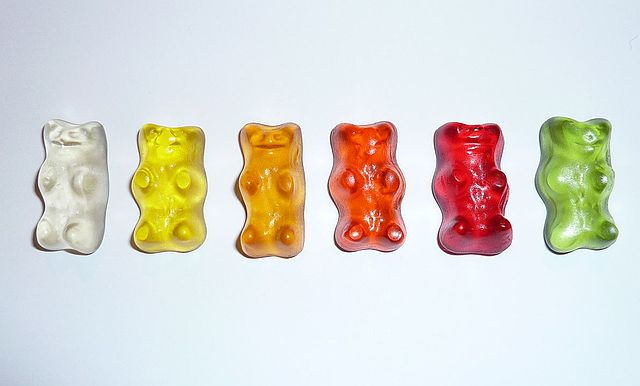# Combinations

Have you ever seen a lotto draw? Let's explore what happens with a simplified version of the game.

In our lotto game, three balls are chosen at random out of a barrel of 20 balls. Let's suppose the balls are numbered $1, 23$ and $36$. It doesn't matter what order they come out of the barrel in. They could arrive in any of the following orders:

• 1, 23, 36
• 1, 36, 23
• 23, 1 ,36
• 23, 36, 1
• 36, 1, 23
• 36, 23, 1
All six orders give us the same set of $3$ numbers. We can't repeat any of the three numbers, but we don't care what order they arrive in.

Our list of 6 possible orders is a list of permutations of these numbers. If you don't remember much about permutations, it might be a good idea to read the article on arrangements of objects in lines.

Our single set of $3$ numbers is an example of what we call a combination. Repetition is not allowed, and order does not matter. By forgetting about the order, we have reduced the number of possible sets of numbers by a factor of $3!$, which is the number of arrangements of the three numbers in a straight line.

This idea gives us a way to calculate the number of combinations of $r$ objects out of a set of $n$ objects. That is, the number of ways of selecting $r$ objects out of a set of $n$ objects, where order does not matter and repetition is not allowed. To calculate how many combinations there are we

1. Work out how many ways there are of arranging $r$ of the $n$ objects in a line without repetition (${}^nP_r$)
2. Divide by the number of ways of arranging the $r$ objects in a line without repetition ($r!$)
So, our total number of combinations is given by the formula
$\dfrac{n!}{(n - r)!} \times \dfrac{1}{r!} = \dfrac{n!}{r!(n - r)!}$

Different mathematicians use different notation for this formula, but they all mean the same thing:

$\dfrac{n!}{(n - r)!} = \begin{pmatrix}n\\r\end{pmatrix} = {}^nC_r = {}_n C_r = C(n,r)$
Each one of them refers to the number of ways of choosing $r$ objects out of a set of $n$ objects, where order does not matter and repetition is not allowed.

### ExampleIn how many different ways can we choose 3 gummi bears out of these 6 gummi bears?

Note: once we've used a gummi bear, we can't replace it and we can't use it again. So, no repetition is allowed.

Solution:

The order of our selection does not matter ( red, green, yellow is the same as red, yellow, green ) and no repetition is allowed, so we are looking for combinations. The total number of selections is

$\begin{pmatrix}6\\3\end{pmatrix} = \dfrac{6!}{3! (6 - 3)!} = \dfrac{6!}{3! 3!} = 20$.

### Fun Facts about the Combinations Formula

The combinations formula is symmetric:

$\dfrac{n!}{r!(n - r)!} = \dfrac{n!} {(n - r)!(n - (n - r))!}$
so,
$\begin{pmatrix}n\\r\end{pmatrix} = \begin{pmatrix} n\\n - r\end{pmatrix}$.

We can read the value of $\begin{pmatrix}n\\r\end{pmatrix}$ from Pascal's triangle: if we number the rows, starting with 0, then $\begin{pmatrix}n\\r\end{pmatrix}$ is the rth entry of row number n.

$\begin{pmatrix}n\\r\end{pmatrix}$ is the number of subsets with $r$ elements of a set of $n$ elements.

## Multi-sets: "Combinations" with Repetition

This is pretty tricky stuff, but it's included here for completeness. Let's suppose you want to allow repetition in your set, but the order of selection still doesn't matter.

For example, you might be making a selection of 20 gummi-bears from a collection of gummi-bears with 6 different colours. If you allow yourself to choose two or more of the same colour, you end up with a multi-set, which is mathematician talk for a set with repeated elements.

How do we go about counting the number of selections of gummi bears now?

Well, we have to think about the problem slightly differently. We have 20 different slots to fill with 6 different colours of lollies. As well as counting the number of copies of each colour, we also need to count the transitions between each colour. If there are 6 colours, there are $6 - 1 = 5$ of these. So, we actually need to select $20$ out of $20 + 6 - 1$ objects. This can be done in

$\begin{pmatrix}20 + 6 -1 \\ 20\end{pmatrix} = \begin{pmatrix}25\\20\end{pmatrix} = 53,130$
ways. In general, the number of ways to select $r$ objects from a set of $n$ objects, where repetition is allowed and order does not matter is given by the formula
$\begin{pmatrix}r + n -1 \\ r\end{pmatrix} = \dfrac{(r + n - 1)!}{r!(n-1)!}$

## Conclusion

Many people find counting very challenging. Don't be discouraged. The best thing you can do is to get lots of practice. Keep trying, but remember:

• Use combinations when order does not matter and repetition is not allowed.
• Use permutations when order matters and repetition is not allowed.

### Description

This chapter series is for Year 10 or higher students, topics include

• Arranging Objects in Lines
• Factorial
• Subsets
• Four colour theorem

and more

### Audience

Year 10 or higher students

### Learning Objectives

These chapters are related to data and in particular "Counting" topics such as Binomial Theorem, Subsets etc

Author: Subject Coach
You must be logged in as Student to ask a Question.